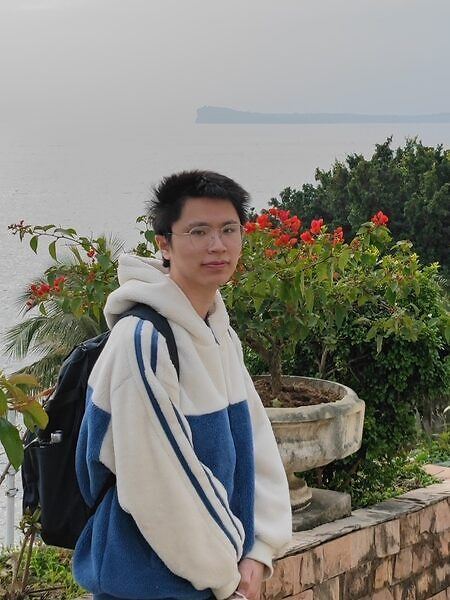# Kinetic Theory: Stability, Regularity and Spectral Analysis of the Boltzmann Equation### Date

Thursday, March 9, 2023 - 13:30 to 15:00

L4E26

### Description

Title: Kinetic Theory: Stability, Regularity and Spectral Analysis of the Boltzmann Equation

Abstract: In this talk, we will study the stability, regularity and spectral analysis of the Boltzmann equation in the context of kinetic theory for collisional particles. We prove that the equation admits a spectral gap in the presence of an inflow boundary condition, introducing a new Hilbert space with an exponential weight function that involves the inner product of space and velocity variables. Our results reveal a new insight of hypocoercivity for kinetic equations with soft potentials, providing exponential decay for solutions in contrast with the sub-exponential decay in either the spatially homogeneous case or the case of torus domain. This talk in based on recent paper [Deng & Duan, Comm. Math. Phys. 397, 1441--1489 (2023)].

All-OIST Category: